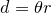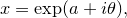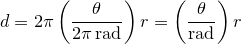## 4.6.1 Treatment of angles in Pyxplot

There are a small number of cases where Pyxplot’s handling of units does not completely follow normal (i.e. SI) conventions, which require further explanation. The most obvious such case is its handling of angles.

By convention, the SI system of units does not have a base unit of angle: instead, the radian is considered to be a dimensionless unit. There are some strong mathematical reasons why this makes sense, since it makes it possible to write equations such asandwhich would otherwise have to be written as, for example,in order to be strictly dimensionally correct.

However, it also has some disadvantages since some physical quantities such as fluxes per steradian are measured per unit angle or per unit solid angle, and the SI system traditionally1 offers no way to dimensionally distinguish these from one another or from quantities with no angular dependence. In addition, many of Pyxplot’s vector graphics commands take rotation angles as inputs, and it is useful to express these in units of angle.

In most cases, the user is free to decide whether angles should have units. All of the following print statements are equivalent:

print sin(pi)
print sin(180*unit(deg))
print sin(0.5*unit(rev))


However, it is useful to be able to define whether inverse trigonometric functions such as asin(x) and atan(x) return results with units of angle, or which are dimensionless. By default, these functions return dimensionless results, but this may be changed using the commands:

set unit angle dimensionless
set unit angle nodimensionless


Note that even when inverse trigonometric functions are set to return dimensionless outputs, expressions such as unit(rad)+1 are still dimensionally incorrect. Functions such as sin(x) and exp(x) can always accept inputs which are either dimensionless, or have units of angle.

Footnotes

1. Radians are sometimes treated in the SI system as supplementary or derived units.